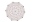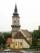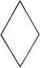# Diagonals

Calculate the length of the diagonals of the rhombus if its side is long 5 and one of its internal angle is 80°.

Result

u1 =  6.43
u2 =  7.66

#### Solution:Leave us a comment of example and its solution (i.e. if it is still somewhat unclear...):

Showing 0 comments:Be the first to comment!#### To solve this example are needed these knowledge from mathematics:

Cosine rule uses trigonometric SAS triangle calculator. See also our trigonometric triangle calculator.

## Next similar examples:

1. Diagonals in diamondIn the rhombus is given a = 160 cm, alpha = 60 degrees. Calculate the length of the diagonals.
2. DiagonalsWhat x-gon has 54 diagonals?
3. Inner anglesThe inner angles of the triangle are 30°, 45° and 105° and its longest side is 10 cm. Calculate the length of the shortest side, write the result in cm up to two decimal places.
4. Side cIn △ABC a=2, b=4 and ∠C=100°. Calculate length of the side c.
5. DiagonalCan a rhombus have the same length diagonal and side?
6. Rotatable towerRotatable tower situated in the city center has ground shape of a regular polygon. If the tower is rotated by 14.4° around its centerpiece it looks from the side same. Your task is to calculate at least how many vertices can have a ground plan view of the.
7. Four sides of trapezoidIn the trapezoid ABCD is |AB| = 73.6 mm; |BC| = 57 mm; |CD| = 60 mm; |AD| = 58.6 mm. Calculate the size of its interior angles.
8. Greatest angleCalculate the greatest triangle angle with sides 197, 208, 299.
9. Angles by cosine lawCalculate the size of the angles of the triangle ABC, if it is given by: a = 3 cm; b = 5 cm; c = 7 cm (use the sine and cosine theorem).
10. DiagonalCan be a diagonal of diamond twice longer than it side?
11. Scalene triangleSolve the triangle: A = 50°, b = 13, c = 6
12. In a 2In a thirteen sided polygon, the sum of five angles is 1274°, four of the eight angles remaining are equal and the other four are 18° less than each of the equal angles. Find the angles. .
13. Reference angleFind the reference angle of each angle:
14. Vector sumThe magnitude of the vector u is 12 and the magnitude of the vector v is 8. Angle between vectors is 61°. What is the magnitude of the vector u + v?
15. AnglesIn the triangle ABC, the ratio of angles is: a:b = 4: 5. The angle c is 36°. How big are the angles a, b?
16. The pondWe can see the pond at an angle 65°37'. Its end points are 155 m and 177 m away from the observer. What is the width of the pond?
17. Theorem proveWe want to prove the sentense: If the natural number n is divisible by six, then n is divisible by three. From what assumption we started?